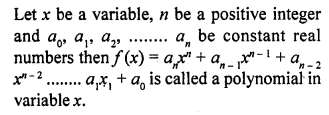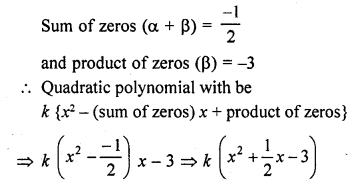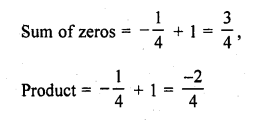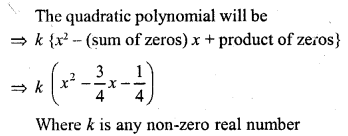• +91 9971497814
• info@interviewmaterial.com

# RD Chapter 2- Polynomials Ex-VSAQS Interview Questions Answers

### Related Subjects

Question 1 : Define a polynomial with real co-efficients.Question 2 : Define degree of a polynomial.

Answer 2 : The exponent of the highest degree term in a polynomial is known as its degree. A polynomial of degree O is called a constant polynomial.

Question 3 : Write the standard form of a linear polynomial with real co-efficients.

Answer 3 : ax + b is the standard form of a linear polynomial with real co-efficients and a ≠ 0

Question 4 : Write the standard form of a quadratic polynomial with real co-efficients.

ax2 + bx + c is astandard form of quadratic polynomial with real co-efficients and a ≠ 0.

Question 5 : Write the standard form of a cubic polynomial with real co-efficients.

ax3 + bx2 + cx + d is a standard form of cubicpolynomial with real co-efficients and a ≠ 0.

Question 6 : Define value of a polynomial at a point.

Answer 6 : If f(x) is a polynomial and a is any real number then the real number obtained by replacing x by α in f(x) is called the value of f(x) at x = α and is denoted by f(α).

Question 7 : Define zero of a polynomial.

Answer 7 : A real number a is a zero of a polynomial f(x) if f(α) = 0.

Question 8 : The sum and product of the zeros of a quadratic polynomial are – 1/2 and -3 respectively. What is the quadratic polynomial ?Question 9 : Write the family of quadratic polynomials having – 1/4 and 1 as its zeros.Question 10 : If the product of zeros of the quadratic polynomial f(x) = x2 – 4x + k is 3, find the value of k.

We know that a quadratic polynomial x2 –(sum of zeros) x + product of zeros
In the given polynomial f(x) = x2 –4x + k is the product of zeros which is equal to 3
k = 3

Todays Deals### RD Chapter 2- Polynomials Ex-VSAQS Contributorskrishan

Name:
Email:

# Latest News# 9000 interview questions in different categories